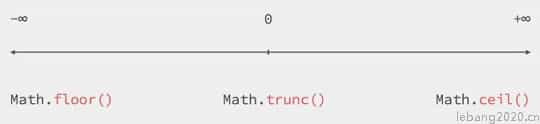﻿ JavaScript中数字的四舍五入和截断 - 乐帮网

# JavaScript中数字的四舍五入和截断

2021-01-07  乐帮网

javascript

3.14159 ≈ 3.1416

3.14159 ≈ 3.1415

JavaScript中对数字的舍入和截取

Math.round() 四舍五入到最接近的整数（如果分数位等于或大于0.5，则四舍五入）
Math.floor() 向下舍取最最接近的整数
Math.ceil() - 向上入取最最接近的整数

``````Math.round(3.14159)  // 3
Math.round(3.5)      // 4
Math.floor(3.8)      // 3
Math.ceil(3.2)       // 4``````

``````Math.round(3.14159 * 100) / 100  // 3.14

3.14159.toFixed(2);              // 3.14 returns a string
parseFloat(3.14159.toFixed(2));  // 3.14 returns a number``````

JavaScript 截断数字

Math.trunc（）只是删除所有小数位。 它采用一个参数，该参数是一个数字。 如果参数为正数，则其结果与Math.floor（）完全相同。 对于负数，它执行效果与Math.ceil（）相同。

``````Math.trunc(3.14159);   //  3
Math.trunc(-3.14159);  // -3``````

Firefox >= 25
Internet Explorer >= Nope :(
Opera >= 25
Safari >= 7.1

``````3.14159 | 0;   //  3
-3.14159 | 0;  // -3

3000000000.1 | 0  // -1294967296``````

Math.round（）-舍入到最接近的整数
Math.floor（）-向下舍入为负无穷大
Math.ceil（）-向上舍入为正无穷大
Math.trunc（）-向上或向下舍入为零（老旧浏览器不支持）